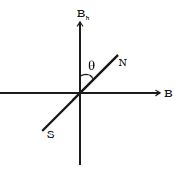# Describe Theory of Tangent Galvanometer

Theory of Tangent GalvanometerWhen the plane of the coil is placed parallel to the horizontal component of Earth’s magnetic induction (Bh) and a current is passed through the coil, there will be two magnetic fields acting perpendicular to each other: (1) the magnetic induction (B) due to the current in the coil acting normal to the plane of the coil and (2) the horizontal component of Earth’s magnetic induction (Bh) (Figure).Due to these two crossed fields, the pivoted magnetic needle is deflected through an angle θ. According to tangent Law,

B = Bh tan θ … 1)

If a current I passes through the coil of n turns and of radius a, the magnetic induction at the centre of the coil is

B = (μ0 NI / 2α) … (2)

Substituting equation (2) in equation (1)

μ0 NI / 2α = Bh tan θ

so, I = (2a Bh / μ0 N) tan θ

I = K tan θ

where K = 2a Bh / μ0 N; is called the reduction factor of the tangent galvanometer. It is a constant at a place. Using this equation, current in the circuit can be determined.

Since the tangent galvanometer is most sensitive to a deflection of 450, the deflection has to be adjusted to be between 300 and 600.